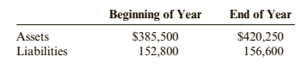Chapter 1, Problem 65APSA### Cornerstones of Financial Accounti...

4th Edition
Jay Rich + 1 other
ISBN: 9781337690881

#### Solutions

Chapter
Section### Cornerstones of Financial Accounti...

4th Edition
Jay Rich + 1 other
ISBN: 9781337690881
Textbook Problem
60 views

# Problem 1-65A Relationships Among Financial StatementsCarson Corporation reported the following amounts for assets and liabilities at the beginning and end of a recent year.Required:Calculate Carson’s net income or net loss for the year in each of the following independent situations: Carson declared no dividends. and its common stock remained unchanged. Carson declared no dividends and issued additional common stock for $40,000 cash. Carson declared dividends totaling$5000 and its common stack remained unchanged. Carson declared dividends totaling $20,000 and issued additional common stock for$35,000.

To determine

Concept Introduction:

Financial statements: Financial statements are prepared to summaries the account at the end of the period. The statements prepared are Income statement, Balance sheet, Statement of owner’s equity and Cash flows statements.

Accounting equation:

Accounting equation represents the mathematical relationship between assets, liabilities and equity. According to this equation, assets are equal to the sum of liabilities and equity. The formal for basic accounting equation is as follows:

Assets = Liabilities + Equity

Statement of Retained earnings:

Items reported on a retained earnings statement are explained as follows:

• Beginning Balance of Retained earnings is the ending balance of the retained earnings of the previous year.
• Net Income or Net loss is the amount of net income earned or net loss incurred for the year.
• Dividends Paid are taken for the year.
• Ending Balance of Retained earnings is calculated as follows:

Ending Balance of Retained earnings = Beginning Balance of Retained earnings + Net Income − Net income − Dividends Paid

To Calculate:

The Net income (loss) under each of the given situation.

Explanation

The Net income (loss) under each of the given situation is calculated as follows:

 Situation 1 Situation 2 Situation 3 Situation 4 Beginning Assets (A) $385,500$ 385,500 $385,500$ 385,500 Beginning Liabilities (B) $152,800$ 152,800 $152,800$ 152,800 Beginning Equity (C) = (A-B) $232,700$ 232,700 $232,700$ 232,700 Ending Assets (D) $420,250$ 420,250 $420,250$ 420,250

### Still sussing out bartleby?

Check out a sample textbook solution.

See a sample solution

#### The Solution to Your Study Problems

Bartleby provides explanations to thousands of textbook problems written by our experts, many with advanced degrees!

Get Started

#### List and describe the different types of databases.

Database Systems: Design, Implementation, & Management

#### What specific information do you need to create a work breakdown structure?

Systems Analysis and Design (Shelly Cashman Series) (MindTap Course List)

#### Convert 10,000 ft-lbf of energy into BTU, Joules, and kilojoules.

Fundamentals of Chemical Engineering Thermodynamics (MindTap Course List)

#### What does the kernel do?

Enhanced Discovering Computers 2017 (Shelly Cashman Series) (MindTap Course List)